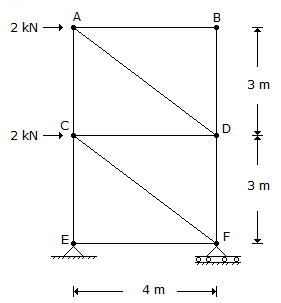# Civil Engineering - UPSC Civil Service Exam Questions - Discussion

29.

A pin-jointed tower truss is loaded as shown in the given figure. The force induced in the member DF is[A]. 1.5 kN (tension) [B]. 4.5 kN (tension) [C]. 1.5 kN (compression) [D]. 4.5 kN (compression)

Explanation:

No answer description available for this question.

 Roland said: (Dec 1, 2013) Answer is D, Member AD is in compression hence at joint D the vertical component of member AD will be balanced by member DF (compression).

 Francis said: (Feb 10, 2014) Yes, it must be compression but I think it would only be 1.5kN.

 Hrisikesh Sil said: (Sep 1, 2015) It should be in compression.

 Daman Sharma said: (Apr 4, 2016) It must be 1.5 compressions. So the answer should be (c).

 Chintu said: (Mar 21, 2017) Taking moment about E we will get reaction at F as 4.5 upwards and hence 4.5 kN comp force in member DF.

 Immu Azm said: (Apr 11, 2017) Answer is D. Reaction Re=4.5 downward now apply zero force member rule force in member EF =0 ( force CE in member AND reaction at E are collinear force in non collinear member must be zero ). Now cut the member X-X passing through CA,CD,FD. NOW TAKE moment about C we will get 4.5 * 4 = DF * 4.

 Immu Azm said: (Apr 11, 2017) Reaction at E 4.5 downward and at F 4.5 upwards.

 Maxp50 said: (Jun 9, 2017) Ans is C. Calculate reactions now go with the method of section cutting member EF CF DF. Now, do summation Fx=0 and Fy=0. Force in DF=-1.5KN i.e. compression.

 Mike Edrad said: (Jan 18, 2018) 1.5 KN Compression because it is towards the joint.

 Bharti Sharma said: (May 10, 2018) 1.5 KN Compression. Calculate horizontal and vertical reactions and then by the method of sections, take equilibrium of horizontal, vertical forces and moments.

 Sharma said: (May 30, 2018) 1.5 Tension. The reaction at F will be 4.5 upward. And taking vertical equilibrium we have; 3/5 FC - FD = 4.5. Now, the force in FC will be compressive only and subtracting force FD is equal to reaction 4.5 which means force FD and reaction is in same direction i.e upward which means the force FD is a Tension force

 Arashdeep Singh said: (Aug 7, 2018) Take a section from mid of CE to mid of DF and calculate moment about joint C. Then, You will get a compressive force of 1.5 kN.

 Civi said: (May 12, 2021) The answer is correct. taking reaction about F. 4xE= (2 x 3) + (2 x 6) E = 18/4. E = 4.5 (compression).